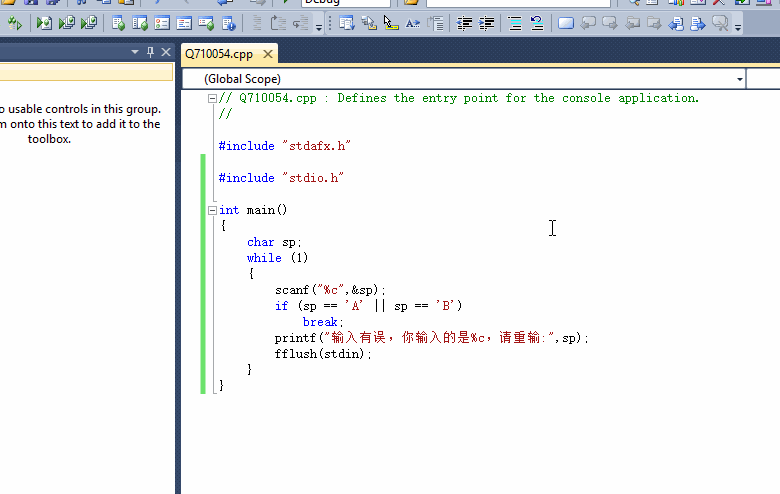C语言中while循环带上scanf语句

char sp；
while (1)
{
scanf("%c",&sp);
if (sp == A || sp == B)
break;
printf("输入有误，请重输:\n,%c",sp);
printf("%c",sp);
}

char sp = ‘c’；
while (1)
{
if (sp == A || sp == B)
break;
printf("输入有误，请重输:\n,%c",sp);
printf("%c",sp);
scanf("%c",&sp);
}

scanf("%c",&sp);
getchar();
while (1)
{
if (sp == 'A' || sp == 'B')
break;
printf("输入有误，请重输:");
scanf("%c",&sp);
getchar();
}

2个回答

``````#include "stdio.h"

int main()
{
char sp;
while (1)
{
scanf("%c",&sp);
if (sp == 'A' || sp == 'B')
break;
printf("输入有误，你输入的是%c，请重输:",sp);
fflush(stdin);
}
}
```````如果问题得到解决，请点我回答左上角的箭头和采纳，谢谢`wmllb521 回复caozhy: 明白了，感谢wmllb521 谢谢老师，我查了很多资料也发现加了getchar（）就没问题了，应该跟 fflush(stdin)是类似的效果吧！`````` void main(){
char sp;
while (1)
{
scanf("%c", &sp);
if (sp == 'A' || sp == 'B')
break;
printf("输入有误，请重输:\n,%c", sp);
printf("%c", sp);
}
return;
}
``````wmllb521 谢谢啦，才发现我的判断字符存在问题while循环的问题，有没有高手指点下？

int main(void) { int x, a, i = 0, k, y; scanf_s("%d",&x); y = x; while (y != 0) { a[i] = y % 2; y = y / 2; i++; } printf("%d = ",x); for (k = i - 1; k >= 0; k--) printf("%1d",a[k]); printf("\n"); getchar(); getchar(); return 0; } while循环过后为什么i=5？ 当x=y=26

C语言编程中循环语句中的输入输出问题

for (i = 0;i < nu;i++) { printf("\n\t\t请输入商品编号:"); scanf("%d", &no); /*检测作品编号是否重复*/ while (p != NULL) { if (strcmp(p->no, no) == 0) { printf( "已经有相同编号的商品"); return; } else { q = p;p = p->next; } } r = (Opi)malloc(sizeof(Opi)); /*创建一个新的文件存储位置*/ r->next = NULL; if (r == NULL) { printf( "分配空间失败!"); return; } if (q == NULL) { head = r; } else { q->next = r; } strcpy(r->no, no); printf("\n\t\t商品名字:"); scanf("%s", r->name); //getchar(); printf("\n\t\t生产厂家:"); scanf("%s", r->ma); //getchar(); printf("\n\t\t售货价格(元):"); scanf("%d", &r->sp); //getchar(); printf("\n\t\t进货价格(元):"); scanf("%d", &r->bid); printf("\n\t\t录入成功!!!!"); } 这个循环语句执行到第二次循环时前三项为啥会同时输出，也就是没法对商品编号，商品名称进行输入

C语言中while语句的意义辨疑

while 后的括号内语句含义是进入循环条件吗？ 如果是，为什么本程序中的while括号内语句表示终止循环条件。 #include <stdio.h> int main (void) { const int secret_code = 13; int code_entered; scanf("%d",&code_entered); while(code_entered !=secret_code) { scanf("%d",&code_entered); } printf("Congratulations!you are cured!\n"); return 0; } 。

#include<stdio.h> #define wage1 8.75 #define wage2 9.33 #define wage3 10.00 #define wage4 11.20 #define rate1 0.15 #define rate2 0.20 #define rate3 0.25 int main(void) { char i; double wage,time,amount; printf("1) \$8.75/hr\n"); printf("2) \$9.33/hr\n"); printf("3) \$10.00/hr\n"); printf("4) \$11.20/hr\n"); printf("5) quit\n"); printf("Enter the number corresponding to desired pay rate or action:"); while(i!='q') { i=getchar(); switch(i) { case 'a':printf("工资为\$%lf,工作时间为:",wage1),wage=wage1;scanf("%lf",&time);break; case 'b':printf("工资为\$%lf,工作时间为:",wage2),wage=wage2;scanf("%lf",&time);break; case 'c':printf("工资为\$%lf,工作时间为:",wage3),wage=wage3;scanf("%lf",&time);break; case 'd':printf("工资为\$%lf,工作时间为:",wage4),wage=wage4;scanf("%lf",&time);break; case 'q':printf("您选择了退出。\n");break; } if(i=='a'||i=='b'||i=='c'||i=='d') { amount=time*wage; printf("税前工资:%.1lf",amount); } if(amount<=300) amount=amount*(1-rate1); if(amount<=450&&amount>300) amount=255+(amount-255)*(1-rate2); if(amount>450) amount=375+(amount-375)*(1-rate3); if(i=='a'||i=='b'||i=='c'||i=='d') printf("税后工资:%.1lf\n",amount); printf("Enter again:"); } }

#include<stdio.h> int main() { int i,j; char n; for(i=0;i<4;i++) for(j=0;j<4;j++) { scanf("%c",&n[i][j]); } printf("\n"); for(i=0;i<4;i++) for(j=0;j<4;j++) { printf("%c",n[i][j]); } return 0; } ![图片说明](https://img-ask.csdn.net/upload/201611/25/1480085985_425991.png)

C语言 while(!feof())似乎陷入了死循环

![图片说明](https://img-ask.csdn.net/upload/202003/17/1584416536_649177.png) 上面是题目，下面这个是我的代码...晕...没办法输出结果，请问要怎么改呢？ ```#include<stdio.h> #include<stdlib.h> int main(){ int s,x,t,max,max1,num,num1; float y; printf("请输入奖金总额：\n"); scanf("%d",&s); num=0; printf("请输入要猜的数额：\n"); while(1){ scanf("%d",&x); max=s-x; max1=abs(max); if(t<max1){ t=max1; num=1; } else if(t==max) num++; num1+=num; } printf("共猜中%d人",num); y=s/num; printf("可分的奖金：%.2f元",y); return 0; } ```

c语言循环语句问题 输出不了结果

if语句没有执行 C语言

CreateList(L,N); temp = L; while(temp->next != temp && temp) { for(i=M-1;i>0;i--) { if(temp->next == NULL) temp->next = L->next; else temp = temp->next; } cout<<temp->next->nData<<endl; temp->next = temp->next->next; } 主要代码如上，断点调试的时候发现if else语句都没有执行，改了一下代码也没有什么作用，求教各位了

C语言中scanf函数为什么无故返回-1？

for 循环中的那三行并排的输入语句 错了吗？

#include <stdio.h> #include <stdlib.h> struct Student { int num; char name; float score; }; int main() { struct Student stu; struct Student t; int i=0,n; int j,k; scanf("%d",&n); for(;i<n;i++) { scanf("%d",&stu[i].num); gets(stu[i].name); scanf("%.2f",&stu[i].score); } for(i=0;i<n-1;i++) { k=i; for(j=i+1;j<n;j++) if(stu[j].score>stu[k].score) k=j; t=stu[k]; stu[k]=stu[i]; stu[i]=t; } for(i=0;i<n;i++) { printf("%3d %5s %6.2f\n",stu[i].num,stu[i].name,stu[i].score); } }

``` #include<stdio.h> #include<stdlib.h> int main() { int month=0; int day=0; int result=0; int days[]={31,28,31,30,31,30,31,31,30,31,30,31}; printf("请输入您选择的月日"); scanf("%d，%d",&month,&day); for(int num=0;num<12;num++) { if(num<month) result+=days[num]; else result+=day; } printf("%d",result); system("pause"); return 0;} ``` 以上是我的全部代码，执行scanf语句的时候，在输入第一个整数后，输入逗号，在输入第二个整数，会吃掉第一个数字，这是为什么呢

#include<stdio.h> int main(void) { float x,y; int n,m,j,i=1; float c; while(~scanf("%f%f",&x,&y)) { n=x; j=y-x+1; int a[j]; int c[j]; while(n<=y) { j=1; m=n*n+n+41; if(i<=m) { a[j]=m; c[j]=a[j]%i; i++; j++; } n=n+1; } if(c[j]!=0) { printf("OK"); } else { printf("Sorry"); } } return 0; } 题目是对于表达式n^2+n+41，当n在（x,y）范围内取整数值时（包括x,y）(-39<=x<y<=50)，判定该表达式的值是否都为素数。 不知道我这样写改怎么去改，才不会WA报错 #include<stdio.h> 代码更新 int main(void) { float x,y; int n,m,j,i=1; j=y-x+1; int a[j]; int c[j]; while(~scanf("%f%f",&x,&y)) { n=x; while(n<=y) { j=1; m=n*n+n+41; if(i<=m) { a[j]=m; c[j]=a[j]%i; i++; j++; } n=n+1; } if(c[j]!=0) { printf("OK"); } else { printf("Sorry"); } } return 0; } ``` 还是报错 ```

C语言小白实现记录学校人员信息：代码如下，为什么在scanf输入第一个人员数据后按回车不能输入下一个人员数据而是程序结束？

``` #include<stdio.h> /*实现记录学校人员信息，包括学生和老师，其中信息包含身份、姓名、性别、职务/班级*/ #include<stdlib.h> struct Position /*结构体变量定义班级或职务*/ { int iClass; char cStaff[]; }; union Membership /*共用体变量定义成员信息*/ { char cStatus[]; char cName[]; int iNumber; char cSex[]; int iGo; /*作为判断是否继续的依据*/ struct Position Text; /*共用体中引用结构体*/ union Membership* pNext; /*指向下一个结点指针*/ }; int iCount; /*定义全局链表长度*/ union Membership* Create() { union Membership *pHead=NULL; /*头指针初始化为空*/ union Membership *pNew, *pEnd; iCount=0; /*链表长度初始化为0*/ pNew=pEnd=(union Membership*)malloc(sizeof(union Membership)); /*创建动态链表*/ printf("请输入学校人员信息:\n"); /*提示信息*/ printf("身份 姓名 编号 性别 职务 班级 是否继续\n"); scanf("%s",&pNew->cStatus); /*输入信息*/ scanf("%s",&pNew->cName); scanf("%d",&pNew->iNumber); scanf("%s",&pNew->cSex); scanf("%d",&pNew->Text.iClass); scanf("%s",&pNew->Text.cStaff); scanf("%d",&pNew->iGo); while(&pNew->iGo!=0); /*判断语句是否进行*/ { iCount++; if(iCount==1) { pNew->pNext=pHead; /*使指向为空*/ pEnd=pNew; /*跟踪新加入的结点*/ pHead=pNew; /*头指针指向首结点*/ } else { pNew->pNext=NULL; /*新结点的指针为空*/ pEnd->pNext=pNew; /*原来的尾结点指向新结点*/ pEnd=pNew; /*pEnd指向新结点*/ } pNew=(union Membership*)malloc(sizeof(union Membership)); /*再次分配节点内存空间*/ scanf("%s",&pNew->cStatus); scanf("%s",&pNew->cName); scanf("%d",&pNew->iNumber); scanf("%s",&pNew->cSex); scanf("%d",&pNew->Text.iClass); scanf("%s",&pNew->Text.cStaff); scanf("%d",&pNew->iGo); } free(pNew); /*释放无用空间*/ return pHead; } void Print(union Membership* pHead) { union Membership *pTemp; /*定义临时指针*/ int iIndex=1; /*定义结点序号*/ printf("数据库中共有%d个人\n",iCount); printf("\n"); pTemp=pHead; /*指针得到首结点地址*/ while(pTemp!=NULL) { printf("第%d个人员信息如下:\n"); printf("身份:%s\n",pTemp->cStatus); printf("姓名:%s\n",pTemp->cName); printf("编号:%d\n",pTemp->iNumber); printf("性别:%s\n",pTemp->cSex); printf("班级:%d",pTemp->Text.iClass); printf("职务:%s\n",pTemp->Text.cStaff); printf("\n"); pTemp=pTemp->pNext; /*移动临时指针到下一个结点*/ iIndex++; } } int main() { union Membership *pHead; /*定义头结点*/ pHead=Create(); /*创建结点*/ Print(pHead); /*输出链表*/ return 0; /*程序结束*/ } ```

2020阿里全球数学大赛：3万名高手、4道题、2天2夜未交卷

HashMap底层实现原理，红黑树，B+树，B树的结构原理 Spring的AOP和IOC是什么？它们常见的使用场景有哪些？Spring事务，事务的属性，传播行为，数据库隔离级别 Spring和SpringMVC，MyBatis以及SpringBoot的注解分别有哪些？SpringMVC的工作原理，SpringBoot框架的优点，MyBatis框架的优点 SpringCould组件有哪些，他们...

《Oracle Java SE编程自学与面试指南》最佳学习路线图2020年最新版（进大厂必备）

《Oracle Java SE编程自学与面试指南》最佳学习路线图（2020最新版）